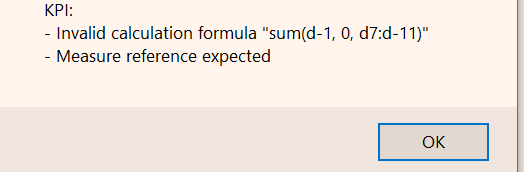# Calculation to Sum all Measures in Row

There's an instance where I need to create a total for multiple different measures all in one row. This formula (sum(d1,0,all)) works initially. But when I close and reopen the report containing that formula, I get this error message. Is there another formula I could try?1 comment
• Hi Taylor,

That is an unexpected and weird behavior, indeed. I have never heard about formulas changing by itself, but maybe this is a first.

Your initial formula, sum(d1, 0, all) should work fine - summing up all measures in a row. If you have five measures, sum(d1, 0, m1:m5), would give the same result.

I don't know what is causing your problem - maybe you can provide a little more details? A screenshot of the crosstab behind the KPI might be helpful.

BR / Ole